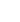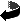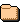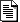```PDS_VERSION_ID        = PDS3
LABEL_REVISION_NOTE   = "
20141031 SwRI:BTCarcich
20160905 SwRI:BTCarcich
20161025 SwRI:BTCarcich
- moved Solar spectrum to document/
"
RECORD_TYPE           = STREAM
OBJECT                = TEXT
INTERCHANGE_FORMAT    = ASCII
PUBLICATION_DATE      = 2019-04-22
NOTE                  = "Description of the contents of the calib dir."
END_OBJECT            = TEXT
END

########################################################################
### N.B. A description of the calibration procedure is in the
###      SOC Instrument Interface Control Document in the
###      /DOCUMENT/ directory of this archive
########################################################################

Notes:

As of 2014-10-31, all in-flight observations have METs greater than
0005257679, the MET of the earliest calibration directory, so the default/
and initial/ directory calibration files are never used.

As of 2014-10-31, the errormap calibration files contain all zeroes in their
first band, and the other band are not used in the calibration.  So all
pixels in the error map extension are zero.

As of 2014-10-31, there are no rollover maps, and the default rollover
scheme is used (subtract 4096 from raw values greater than 3850).

As of 2014-10-31, all calibration file maps, except for the electronics
induced readout signal map elecmap.fit, are copied into one of the
extensions of the calibrated data output file.

The per-pixel calibration formula is

C  = (((S - E ) / F ) - O ) * G  / (I * W  * aOmega * gCorr)
i       i    i     i     i     i        i

Where
i is the pixel index subscript, indicating the operation is per-pixel
- that is, all quantities with a subscript i in the formula above
refer to a pixel (LINE,LINE_SAMPLE) from one of the following:
- the raw image input pixel (S), all bands
- the corresponding calibrated image output pixel (O), all bands
- the corresponding pixel from one of the calibration file maps
- All image, and all map dimensions are [256,256] or [256,256,N]
where N refers to the number of image bands (PDS) or planes (FITS)
- PDS bands are numbered from one to N
- FITS image planes are numbered from zero to (N-1)
- PDS band 1 is the same as FITS plane 0
- PDS band N is the same as FITS plane (N-1)
C is the calibrated output pixel value, erg/s/cm^2/Angstrom/sr
S is the raw input pixel value, after correcting for rollover
E is the electronics induced readout signal map pixel value, elecmap
F is the flat field map pixel value, flatmap.fit
O is the offset map pixel value, calmap.fit, second band
G is the gain map pixel value, calmap.fit, first band
gCorr = 0.25, gain correction factor
I is the intergration time, seconds
W is the wavelength width map pixel value, wavemap.fit, second band
aOmega = 0.004*0.004*PI/((2*8.6)*(2*8.6)), pixel solid angle

[calib] Directory containing calibration files for LEISA data.
|
+--  directory for use after MET 0005257679
|   |
|   +-- anglemap.fit  FOV pointing vector for each pixel in the LEISA array
|   +-- calmap.fit  gain and offset for each pixel in the LEISA array
|   +-- elecmap.fit offset value for each pixel in the LEISA array
|   +-- errormap_v0.fit baseline array for storing of signal error values
|   +-- flatmap.fit flatfield value for each pixel in the LEISA array
|   +-- pixelmap.fit  file containing map of LEISA bad pixels
|   +-- wavemap.fit center wavelength and filter width of each LEISA pixel
|
+--  directory for use after MET 0019690000
|   |
|   +-- anglemap.fit  FOV pointing vector for each pixel in the LEISA array
|   +-- calmap.fit  gain and offset for each pixel in the LEISA array
|   +-- elecmap.fit offset value for each pixel in the LEISA array
|   +-- errormap_v0.fit baseline array for storing of signal error values
|   +-- flatmap.fit  flatfield value for each pixel in the LEISA array
|   +-- pixelmap.fit  file containing map of LEISA bad pixels
|   +-- wavemap.fit center wavelength and filter width of each LEISA pixel
|
+--  directory for use after MET 0030594839
|   |
|   +-- anglemap.fit  FOV pointing vector for each pixel in the LEISA array
|   +-- calmap.fit  gain and offset for each pixel in the LEISA array
|   +-- elecmap.fit offset value for each pixel in the LEISA array
|   +-- errormap_v0.fit baseline array for storing of signal error values
|   +-- flatmap.fit flatfield value for each pixel in the LEISA array
|   +-- pixelmap.fit   file containing map of LEISA bad pixels
|   +-- wavemap.fit center wavelength and filter width of each LEISA pixel
|
+-- calinfo.txt  This file
|
+-- [default] Default cal files for LEISA, if MET or initial files missing
|   |
|   +-- anglemap.fit   FOV pointing vector for each pixel in the LEISA array
|   +-- calmap.fit  gain and offset for each pixel in the LEISA array
|   +-- elecmap.fit offset value for each pixel in the LEISA array
|   +-- errormap_v3.fit baseline array for storing of signal error values
|   +-- flatmap.fit  flatfield value for each pixel in the LEISA array
|   +-- pixelmap.fit   file containing map of LEISA bad pixels
|   +-- wavemap.fit center wavelength and filter width of each LEISA pixel
|
+-- [deliv_20160331] Cal files for LEISA, delivered with Pluto data
|   |
|   +-- anglemap.fit   FOV pointing vector for each pixel in the LEISA array
|   +-- bondjointflaringmaskmap.fit  Map of LEISA pixels in flaring region
|   +-- calmap.fit  gain and offset for each pixel in the LEISA array
|   +-- elecmap.fit offset value for each pixel in the LEISA array
|   +-- flatmap.fit  flatfield value for each pixel in the LEISA array
|   +-- pixelmap.fit   file containing map of LEISA bad pixels
|   +-- wavemap.fit center wavelength and filter width of each LEISA pixel
|
+-- [initial] directory for use from launch until MET 0005257679 pre-flight
|
+-- anglemap.fit   FOV pointing vector for each pixel in the LEISA array
+-- calmap.fit  gain and offset for each pixel in the LEISA array
+-- elecmap.fit offset value for each pixel in the LEISA array
+-- errormap_v3.fit baseline array for storing of signal error values
+-- flatmap.fit  flatfield value for each pixel in the LEISA array
+-- pixelmap.fit   file containing map of LEISA bad pixels
+-- wavemap.fit center wavelength and filter width of each LEISA pixel
```
```Name                                    Last modified      Size  DescriptionParent Directory                                             -0005257679/                             20-May-2019 19:42    -0019690000/                             20-May-2019 19:42    -0030594839/                             20-May-2019 19:42    -default/                                20-May-2019 19:42    -deliv_20160331/                         20-May-2019 19:42    -initial/                                20-May-2019 19:42    -calinfo.txt                             20-May-2019 19:42   10K
```
Apache/2.2.15 (Scientific Linux) Server at pdssbn.astro.umd.edu Port 443